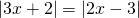Chapter 2: Linear Equations

# 2.5 Absolute Value Equations

When solving equations with absolute values, there can be more than one possible answer. This is because the variable whose absolute value is being taken can be either negative or positive, and both possibilities must be accounted for when solving equations.

Example 2.5.1

Solve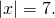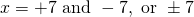When there are absolute values in a problem, it is important to first isolate the absolute value, then remove the absolute value by considering both the positive and negative solutions. Notice that, in the next two examples, all the numbers outside of the absolute value are moved to one side first before the absolute value bars are removed and both positive and negative solutions are considered.

Example 2.5.2

Solve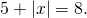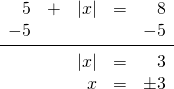Example 2.5.3

Solve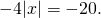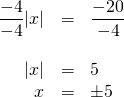Note: the objective in solving for absolute values is to isolate the absolute value to yield a solution.

Example 2.5.4

Solve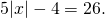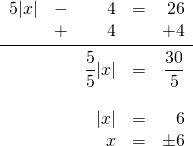Often, the absolute value of more than just a variable is being taken. In these cases, it is necessary to solve the resulting equations before considering positive and negative possibilities. This is shown in the next example.

Example 2.5.5

Solve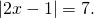Since absolute value can be positive or negative, this means that there are two equations to solve.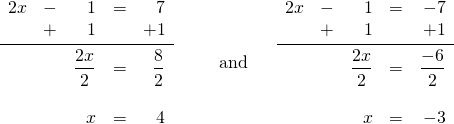Remember: the absolute value must be isolated first before solving.

Example 2.5.6

Solve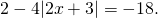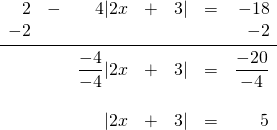Now, solve two equations to get the positive and negative solutions: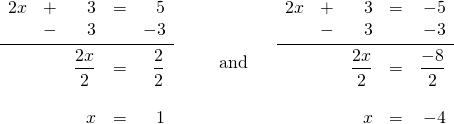There exist two other possible results from solving an absolute value besides what has been shown in the above six examples.

Example 2.5.7

Consider the equation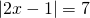from Example 2.5.5.
What happens if, instead, the equation to solve is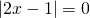or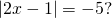For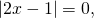there is no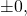so there will be just one solution instead of two. Solving this equation yields: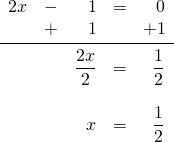For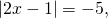the result will be “no solution” or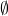, since an absolute value is never negative.

One final type of absolute value problem covered in this chapter is when two absolute values are equal to each other. There will still be both a positive and a negative result—the difference is that a negative must be distributed into the second absolute value for the negative possibility.

Example 2.5.8

Solve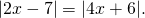Solving this means solving: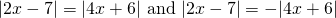Removing the absolute value signs leaves: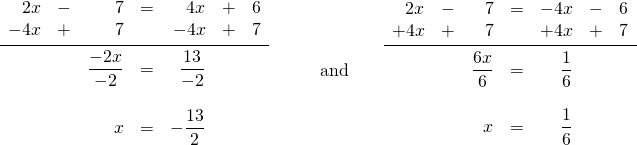# Questions

For questions 1 to 24, solve each absolute value equation.

1.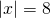2.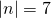3.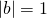4.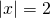5.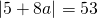6.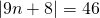7.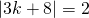8.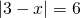9.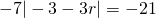10.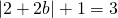11.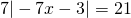12.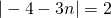13.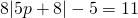14.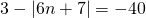15.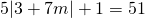16.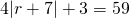17.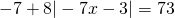18.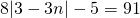19.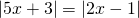20.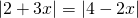21.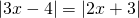22.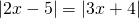23.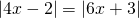24.